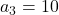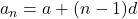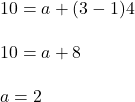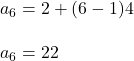## An arithmetic sequence has a common difference of 4 and the 3rd term is 10. What is the 6th term?

Question

An arithmetic sequence has a common difference of 4 and the 3rd term is 10. What is the 6th term?

in progress 0
6 months 2021-07-30T08:26:18+00:00 1 Answers 17 views 0

22

Step-by-step explanation:

Given that,

Common difference, d = 4

3rd term,nth term of an AP is given by :, a is first term

For 3rd term,Now 6th term is given by :Hence, the 6th term is 22.